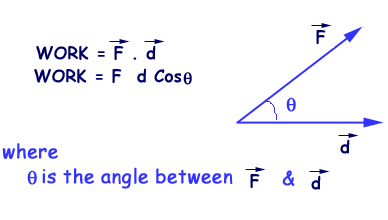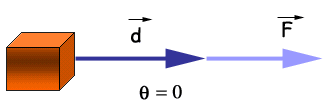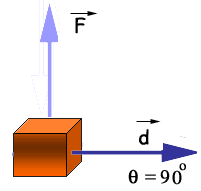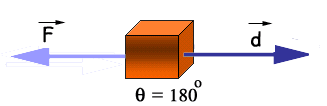# Work

Physical definition of work
“Work is said to be done if a force causes a displacement
in a body in the direction of force”.
OR
“The work done by a constant force is defined as the product of
the component of the force and the displacement in the direction of displacement.”

Mathematical definition
“Work is the scalar product of force and displacement”. OR
“Work is the dot product of force and displacement”.Work is a scalar quantity.

Unit of works

• In S.I system: Joule (j)
• In C.G.S. system: Erg
• In F.P.S. system: ft X lb

Categories of work

1. Positive work:
If force and displacement are in the same direction, work will be positive or if q = 0 orq< 90°2. Zero work:
If force and displacement are perpendicular to each other, work will be zero. i.e.
since q = 90°
Work = 0
as
Work = Fd Cos q
Work = Fd Cos 90°
Work = (F)(d)(0)
Work = 03. Negative work:
In force and displacement are in the opposite direction, work will be negative.Since q = 180°
Work = – ve
as
Work = Fd Cos q
Work = Fd Cos 180°
Work = (F)(d)(-1)
Work = -Fd